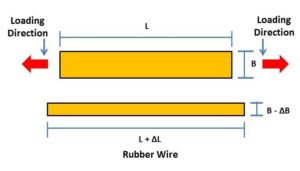# Elastic Constant : Bulk Modulus, Modulus of Rigidity and Poisson’s Ratio

Sharing is Caring :)-

Hello friends.

Today we are going to learn about some important definition used in study of SOM like bulk modulus of elasticity, modulus of rigidity and Poisson’s ratio. In our previous articles, we have learnt about strain, stress and their types and relationship. We have also discussed about young modulus of elasticity in my last post. This article gives you brief information about other three constant.

Let’s start the discussion.

# Elastic Constant:

### Bulk Modulus of Elasticity:

The ratio of direct stress to linear strain under elastic limit is known as young modulus of elasticity. It is a one dimensional constant which gives information about change in linear dimension under loading condition. But when a body undergoes any force, both its linear as well as lateral dimensions will change accordingly. This will change overall dimension or volume of the object.

The change in volume to its original volume under loading condition is known as volumetric strain and the ratio of direct stress to volumetric strain is known as bulk modulus of elasticity. It is define by symbol K.### Modulus of Rigidity:

When we applied shear load (parallel to the object) on an object, the linear dimensions of the objects remain same but the shape of the body deform. The strain associated with the shear stress in known as shear strain. The ratio of shear stress to shear strain is known as modulus of Rigidity. This constant is represented by symbol G.### Poisson’s Ratio:

It is another most useful constant in study of strength of material. We have discussed about lateral strain and linear stain in our previous article.

The ratio of change in dimension to original dimension in direction of applied force is known as linear strain or longitudinal strain and the ratio of change in dimension to original dimension in direction perpendicular to the applied force is known as lateral strain.The ratio of lateral strain to linear strain is known as Poisson’s Ratio. It is represent by μ.This is basic introduction of bulk modulus of elasticity, modulus of rigidity and Poisson’s Ratio. If you have any query regarding this article, ask by commenting. Subscribe our website for more articles regarding strength of material. Thanks for reading it.

### Related Post

Sharing is Caring :)-

This site uses Akismet to reduce spam. Learn how your comment data is processed.